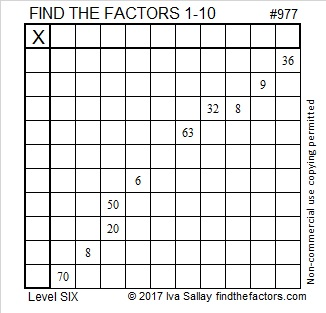# 977 and Level 6

Can you find the logic that is needed to make these ten clues become a multiplication table with the numbers 1 to 10 in the first column and again in the top row? This is a level 6 puzzle so it should be a little bit of a challenge even for adults. Nevertheless, it probably won’t require too much of your time to solve. Go ahead. Give it a try!Print the puzzles or type the solution in this excel file: 10-factors-968-977

Prime number 977 is the sum of nine consecutive prime numbers:
89 + 97 + 101 + 103 + 107 + 109 + 113 + 127 + 131 = 977

31² + 4² = 977 making 977 the hypotenuse of a Pythagorean triple:
248-945-977 calculated from 2(31)(4), 31² – 4², 31² + 4²

• 977 is a prime number.
• Prime factorization: 977 is prime.
• The exponent of prime number 977 is 1. Adding 1 to that exponent we get (1 + 1) = 2. Therefore 977 has exactly 2 factors.
• Factors of 977: 1, 977
• Factor pairs: 977 = 1 × 977
• 977 has no square factors that allow its square root to be simplified. √977 ≈ 31.256999

How do we know that 977 is a prime number? If 977 were not a prime number, then it would be divisible by at least one prime number less than or equal to √977 ≈ 31.3. Since 977 cannot be divided evenly by 2, 3, 5, 7, 11, 13, 17, 19, 23, 29 or 31, we know that 977 is a prime number.Here’s another way we know that 977 is a prime number: Since its last two digits divided by 4 leave a remainder of 1, and 31² + 4² = 977 with 31 and 4 having no common prime factors, 977 will be prime unless it is divisible by a prime number Pythagorean triple hypotenuse less than or equal to √977 ≈ 31.3. Since 977 is not divisible by 5, 13, 17, or 29, we know that 977 is a prime number.

This site uses Akismet to reduce spam. Learn how your comment data is processed.